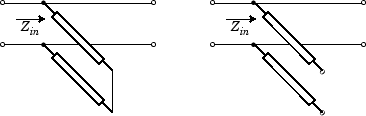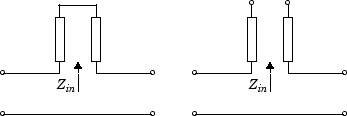# rfckt.rlcgline

Passive component or network

## Description

Use the `rfckt.rlcgline` object to create a RLCG transmission line that is characterized by line loss, line length, stub type, and termination.

Note

`txlineRLCGLine` is recommended over `rfckt.rlcgline` because it enables you to:

• Create a RLCG transmission line that is characterized by line loss, line length, stub type, and termination.

• Build a `circuit` object with a RLCG transmission line.

• Model a RLCG transmission line element in an RF chain created using an `rfbudget` object or the RF Budget Analyzer app, and then export this element to RF Blockset™ or to `rfsystem` System object™ for circuit envelope analysis.

(since R2023b)

## Creation

### Syntax

``h = rfckt.rlcgline``
``h = rfckt.rlcgline(Name,Value)``

### Description

example

````h = rfckt.rlcgline` returns an RLCG transmission line object whose properties are set to their default values.```
````h = rfckt.rlcgline(Name,Value)` sets properties using one or more name-value pairs. For example, `rfckt.rlcgline('LineLenght',0.04)` creates a RLGC transmission line with a physical length of 0.04 meters. You can specify multiple name-value pairs. Enclose each property name in a quote. Properties not specified retain their default values.```

## Properties

expand all

Computed S-parameters, noise figure, OIP3, and group delay values, specified as `rfdata.data` object. `AnalyzedResult` is a read-only property. For more information refer, .Algorithms.

Data Types: `function_handle`

Resistance values per length, specified as a vector in ohms per meter. The resistance values correspond to the frequency values in `'Freq'` property. All values must be positive. The default value is `0`.

Data Types: `double`

Capacitance values per length, specified as a vector in farads per meter. The capacitance values correspond to the frequency values in `'Freq'` property. All values must be positive. The default value is `0`.

Data Types: `double`

Frequency data for the RLCG values, specified as a M-element vector. The values must be positive and correspond to the order of the RLCG values. The default value is `1e9`.

Data Types: `double`

Conductance values per length, specified as a vector in Siemens per meter. The conductance values correspond to the frequency values in `'Freq'` property. All values must be positive. The default value is `0`.

Data Types: `double`

Interpolation method used in `rfckt.rlcgline`, specified as one of the following values:

MethodDescription
`Linear`Linear interpolation
`Spline`Cubic spline interpolation
`Cubic`Piecewise cubic Hermite interpolation

Data Types: `char`

Inductance values per length, specified as vector in henries per meter. The inductance values correspond to the frequency values in `'Freq'` property. All values must be positive. The default value is `0`.

Data Types: `double`

Physical length of transmission line, specified as a scalar in meters. The default value is `0.01`.

Data Types: `double`

Object name, specified as a `1-by-N` character array. `Name` is a read-only property.

Data Types: `char`

Number of ports, specified as a positive integer. `nport`t is a read-only property. The default value is `2`.

Data Types: `double`

Type of stub, specified as one of the following values: `'NotaStub'`, `'Series'`, `'Shunt'`.

Data Types: `double`

Stub transmission line termination, specified as one of the following values: `'NotApplicable'`, `'Open'`, `'Short'`.

Data Types: `double`

## Object Functions

 `analyze` Analyze RFCKT object in frequency domain `calculate` Calculate specified parameters for rfckt objects or rfdata objects `circle` Draw circles on Smith Chart `extract` Extract specified network parameters from rfckt object or data object `listformat` List valid formats for specified circuit object parameter `listparam` List valid parameters for specified circuit object `loglog` Plot specified circuit object parameters using log-log scale `plot` Plot circuit object parameters on X-Y plane `plotyy` Plot parameters of RF circuit or RF data on X-Y plane with two Y-axes `getop` Display operating conditions `polar` Plot specified object parameters on polar coordinates `semilogx` Plot RF circuit object parameters using log scale for x-axis `semilogy` Plot RF circuit object parameters using log scale for y-axis `smith` Plot circuit object parameters on Smith chart `write` Write RF data from circuit or data object to file `getz0` Calculate characteristic impedance of RFCKT transmission line object `read` Read RF data from file to new or existing circuit or data object `restore` Restore data to original frequencies `getop` Display operating conditions `groupdelay` Group delay of S-parameter object or RF filter object or RF Toolbox circuit object

## Examples

collapse all

Create an RLCG transmission line using `rfckt.rlcgline`.

`rlcgtx=rfckt.rlcgline('R',0.002,'C',8.8542e-12,'L',1.2566e-6,'G',0.002')`
```rlcgtx = rfckt.rlcgline with properties: Freq: 1.0000e+09 R: 0.0020 L: 1.2566e-06 C: 8.8542e-12 G: 0.0020 IntpType: 'Linear' LineLength: 0.0100 StubMode: 'NotAStub' Termination: 'NotApplicable' nPort: 2 AnalyzedResult: [] Name: 'RLCG Transmission Line' ```

## Algorithms

The `analyze` method treats the transmission line, which can be lossy or lossless, as a 2-port linear network. It uses the interpolation method you specify in the `IntpType` property to find the R, L, C, and G values at the frequencies you specify when you call `analyze`. Then, it calculates the characteristic impedance, Z0, phase velocity, PV, and loss using these interpolated values. It computes the `AnalyzedResult` property of a stub or as a stubless line using the data stored in the `rfckt.rlcgline` object properties as follows:

• If you model the transmission line as a stubless line, the `analyze` method first calculates the ABCD-parameters at each frequency contained in the modeling frequencies vector. It then uses the `abcd2s` function to convert the ABCD-parameters to S-parameters.

The `analyze` method calculates the ABCD-parameters using the physical length of the transmission line, d, and the complex propagation constant, k, using the following equations:

`$\begin{array}{l}A=\frac{{e}^{kd}+{e}^{-kd}}{2}\\ B=\frac{{Z}_{0}*\left({e}^{kd}-{e}^{-kd}\right)}{2}\\ C=\frac{{e}^{kd}-{e}^{-kd}}{2*{Z}_{0}}\\ D=\frac{{e}^{kd}+{e}^{-kd}}{2}\end{array}$`

Z0 and k are vectors whose elements correspond to the elements of f, the vector of frequencies specified in the `analyze` input argument `freq`. Both can be expressed in terms of the resistance (R), inductance (L), conductance (G), and capacitance (C) per unit length (meters) as follows:

`$\begin{array}{c}{Z}_{0}=\sqrt{\frac{R+j2\pi fL}{G+j2\pi fC}}\\ k={k}_{r}+j{k}_{i}=\sqrt{\left(R+j2\pi fL\right)\left(G+j2\pi FC\right)}\end{array}$`
• If you model the transmission line as a shunt or series stub, the `analyze` method first calculates the ABCD-parameters at the specified frequencies. It then uses the `abcd2s` function to convert the ABCD-parameters to S-parameters.

When you set the `StubMode` property to `'Shunt'`, the 2-port network consists of a stub transmission line that you can terminate with either a short circuit or an open circuit as shown in the following figure.Zin is the input impedance of the shunt circuit. The ABCD-parameters for the shunt stub are calculated as:

`$\begin{array}{c}A=1\\ B=0\\ C=1/{Z}_{in}\\ D=1\end{array}$`

When you set the `StubMode` property to `'Series'`, the 2-port network consists of a series transmission line that you can terminate with either a short circuit or an open circuit as shown in the following figure.Zin is the input impedance of the series circuit. The ABCD-parameters for the series stub are calculated as:

`$\begin{array}{c}A=1\\ B={Z}_{in}\\ C=0\\ D=1\end{array}$`

The `analyze` method uses the S-parameters to calculate the group delay values at the frequencies specified in the `analyze` input argument `freq`, as described in the `analyze` reference page.

 Ludwig, R. and P. Bretchko, RF Circuit Design: Theory and Applications, Prentice-Hall, 2000